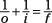##### Optics For Dummies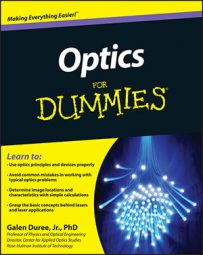Imaging is a key function of optics. Specific optics equations can help you determine the basic characteristics of an image and predict where it will form. Use the following optics equations for your imaging needs:

• Lateral magnification: Lateral magnification is one way you can describe how big the image is compared to the original object. Here are the equations: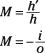• Locating images formed by mirrors: An object placed a certain distance away from a mirror will produce an image at a certain distance from the mirror. In some cases where the mirrors are curved, you may be given the focal length of a mirror. Use these equations: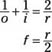• Location of images formed by a refracting surface: An object placed a certain distance away from a refracting surface will produce an image at a certain distance from the surface. The equation for this is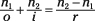• The lens maker's formula: This equation allows you to calculate the focal length of a lens if all you know is the curvature of the two surfaces. Here's the lens maker's formula: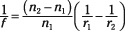• The thin lens equation: An object placed a certain distance away from a lens will produce an image at a certain distance from the lens, and the thin lens equation relates the image location to the object distance and focal length. The following is the thin lens equation: